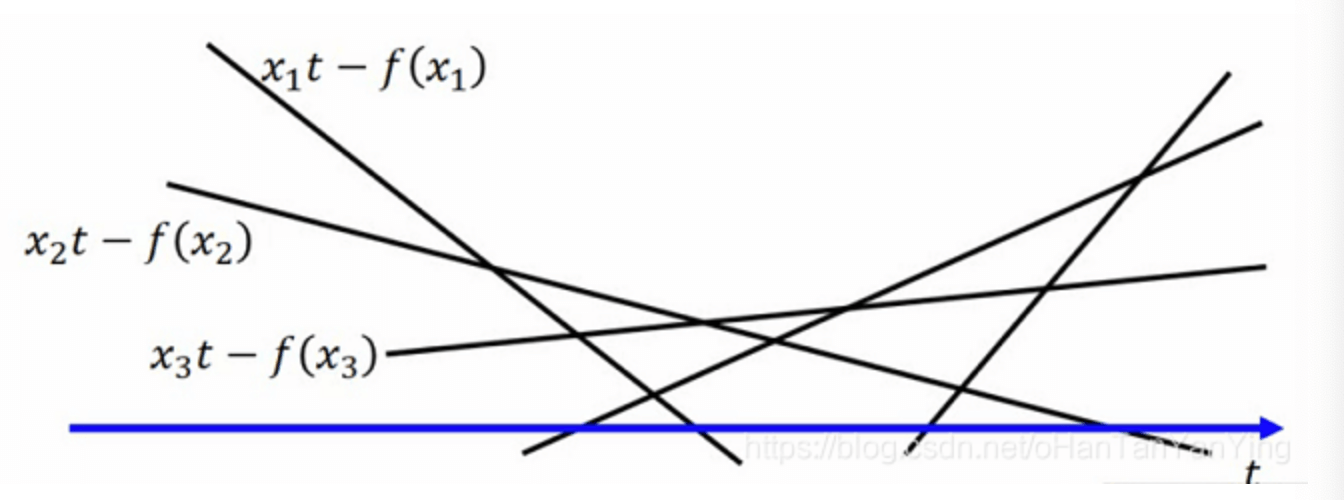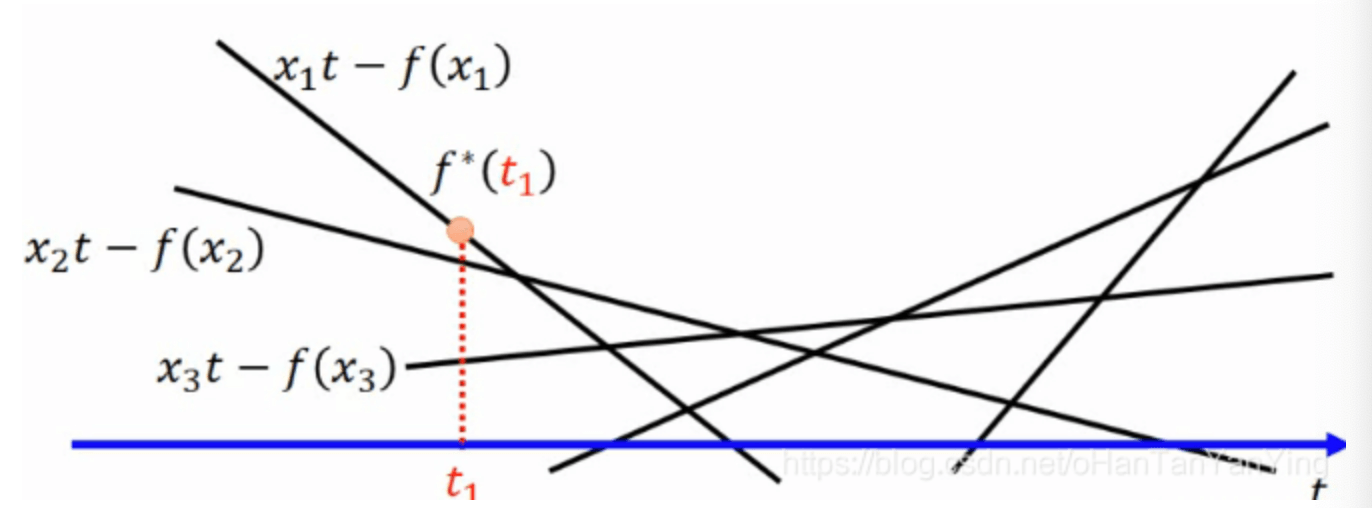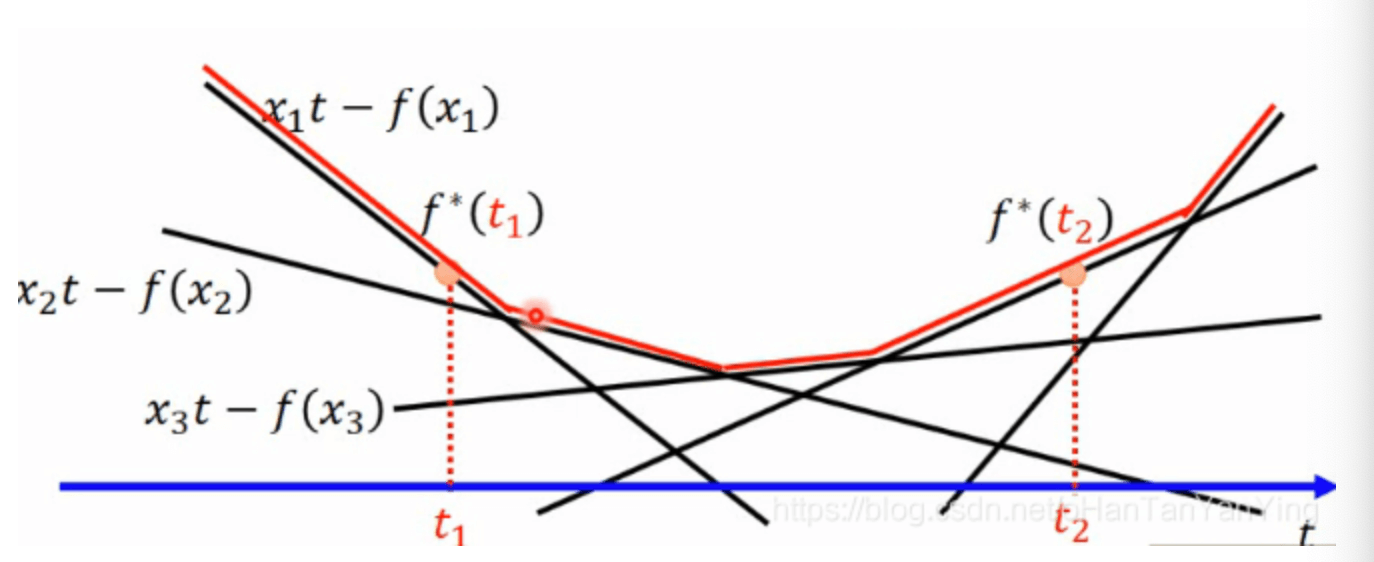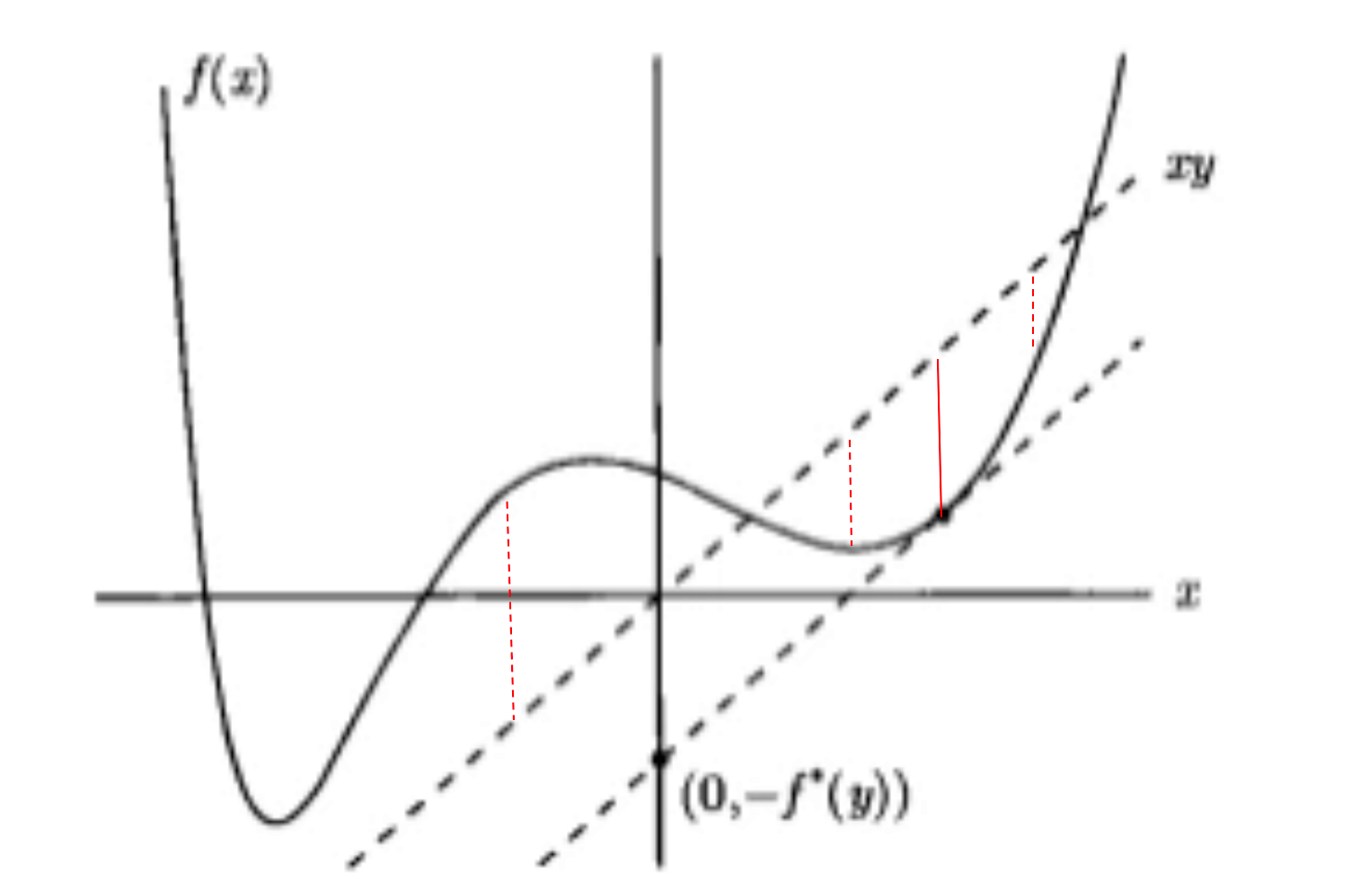# 【数学基础】第十九课：凸优化进阶

## 共轭函数，共轭函数的性质，对偶函数，对偶性

Posted by x-jeff on April 11, 2021

# 1.共轭函数

$f^*(y)=\sup \limits_{x \in dom \ f} (y^Tx-f(x))$

$x\in dom \ f$表示$x$在$f$的定义域内取值。

$f^*(t)=\max \limits_{x \in dom \ (f)} \{xt-f(x) \}$

1. 共轭函数$f^*$是一个凸函数。
2. 如果$g$是$f$的凸闭包，那么$g^{*}=f^{*}$。
3. 对一般的函数$f$，$f^{**} \leqslant f$。
4. 如果$f$是一个凸函数，那么$f^{**}=f$。
5. Fenchel不等式（当$f$可微时亦称为Young不等式）：$f(x)+f^*(y) \geqslant x^T y$。
6. 如果$f$是凸函数而且可微，那么$f^{*}(y)=x^{* T} \nabla f(x^{*})-f(x^{*})$，其中$x^{*}$满足$\nabla f(x^{*})=y$。
7. 如果$g(x)=f(Ax+b)$，则$g^{*}(y)=f^{*}(A^{-T}y)-b^TA^{-T}y$。
8. 如果$f(u,v)=f_1(u)+f_2(v)$，那么$f^{*}(w,z)=f^{*}_1(w)+f^{*}_2(z)$。

## 1.1.性质1的证明

$f^*(t)=\max \limits_{x \in dom \ (f)} \{xt-f(x) \}$## 1.2.性质2的证明## 1.3.性质3的证明

$f^*(y)=\sup \limits_{x \in dom \ f} (y^Tx-f(x))$ $f^*(y) \geqslant (y^Tx-f(x))$ $f(x) \geqslant y^T x -f^*(y)$

$f^*(y)=\sup \limits_{z \in dom \ f} (y^Tz-f(z))$ $f^*(y) \geqslant (y^Tz-f(z))$ $f(z) \geqslant y^Tz - f^*(y)$

$y^Tz$和$z^Ty$是相等的，都是一个数字（即$1\times 1$维）。因此：

$f^{**}(z)=\sup \limits_{y \in dom \ f^*} (z^Ty-f^*(y))$ $f^{**}(z) \leqslant f(z)$

## 1.4.性质4的证明

$f^*(t)=\max \limits_{x \in dom \ (f)} \{xt-f(x) \} \tag{1.4.1}$

$(xt-f(x))'=0 \tag{1.4.2}$

$t=f'_x(x) \tag{1.4.3}$

$f’_x(x)$指的是$f(x)$对$x$求导。如果下标为$t$，则指的是对$t$求导。

$f^*(t)=xt-f(x) \ | \ f'_x(x)=t \tag{1.4.4}$

$f^*(t)$的共轭函数如下：

$f^{**}(s) = \max \limits_{t \in dom \ (f^*)} \{ ts-f^*(t) \} \tag{1.4.5}$

$(ts-f^*(t))_t'=s-(f^*(t))'_t=0 \tag{1.4.6}$

$s=(f^*(t))'_t=x+ tx'_t - f'_x(x) x'_t \tag{1.4.7}$

$s=(f^*(t))'_t=x+tx'_t-tx'_t=x \tag{1.4.8}$

$f^{**}(s)=tx-f^*(t) \tag{1.4.9}$

$f^{**}(s)=tx-f^*(t)=tx-(xt-f(x))=f(x)=f(s) \tag{1.4.10}$

## 1.5.性质6的证明

$\nabla f(x)=y$

$f^*(y)=y^Tx-f(x)=(\nabla f(x))^Tx-f(x)$

$f^*(y)=(\nabla f(x^*))^Tx^*-f(x^*)$

$f^*(y)=x^{*T} \nabla f(x^*)-f(x^*)$

## 1.6.共轭函数的例子

$f^*(y)=\sup \limits_{x \in dom \ f} (y^Tx-x\ln x)$

$\frac{d}{dx} (y^Tx-x\ln x)=y^T-1-\ln x$

$\ln x=y^T-1$ $x=e^{y^T-1}$

$f^*(y)=y^T e^{y^T-1} - e^{y^T-1} \ln (e^{y^T-1})=e^{y^T-1}$

# 2.拉格朗日对偶函数

• 最小化：$f_0(x)$
• 不等条件：$Ax \leqslant b$
• 等式条件：$Cx=d$

\begin{align} g(\lambda,v) & = \inf \limits_{x} (f_0(x) + \lambda^T (Ax-b) + v^T(Cx-d)) \\ & = -b^T \lambda - d^T v + \inf \limits_{x} (f_0(x) + (A^T\lambda + C^T v)^T x ) \\ &= -b^T \lambda - d^T v - f^*_0 (-A^T\lambda - C^T v ) \end{align}

\begin{align} \inf (y^Tx+f_0(x)) &= -\sup (-y^Tx-f_0(x)) \\&= -f^*(-y) \end{align}

$dom \ g=\{(\lambda,v): -A^T\lambda - C^T v \in dom \ f^*_0 \}$

# 3.对偶性

## 3.1.条件1

• 最小化：$f_0(x)$
• 不等条件：$f_i(x) \leqslant b,i=1,\cdots,m$
• 等式条件：$h_i(x)=0,i=1,\cdots,p$

# 4.参考资料